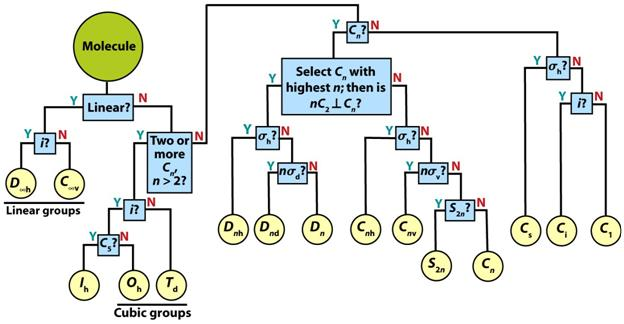# What is the symmetry of the cuboctahedron (FCC metal)?

The background to this is that I've recently given a tutorial wherein we had to go through the determination of point groups for atoms in various lattices (BCC, FCC/CCP and HCP). BCC and HCP, I have no problem with. However, I've now acquired a copy of the lecturer's "official" solutions and there's a bit of discrepancy with the FCC, that I can't fathom: I get an $$O_\mathrm{h}$$ point group, while he gives a $$D_\mathrm{3d}$$ point group.

Here goes:

The coordination geometry of an atom in the FCC structure (assuming one atom/lattice point and only one species of atom, as in an FCC metal) is a cuboctahedron:,By my reckoning we have $$3$$ $$C_4$$ axes, one through each pair of square faces, and a $$C_3$$ through each pair of triangular faces.

Following the classic flowchart:The number of high-symmetry rotational axes is surely enough to exclude $$D_\mathrm{3d}$$ already?

The only thing that makes me think that the lecturer hasn't just made an error is this line from the Wikipedia page for the cuboctahedron:

With $$O\mathrm{h}$$ symmetry, order $$48$$, it is a rectified cube or rectified octahedron (Norman Johnson).

With $$T_\mathrm{d}$$ symmetry, order $$24$$, it is a cantellated tetrahedron or rhombitetratetrahedron.

With $$D_\mathrm{3d}$$ symmetry, order $$12$$, it is a triangular gyrobicupola.

This is no doubt due to deficiencies in my understanding of group theory, but I don't understand how the same shape can simultaneously have multiple point groups, nor how you would select which one is relevant to a particular analysis.

• I will update the question to make this clearer, but the assumption when solving was that we had an FCC metal structure, ie. only one atom. The Wikipedia quote was from a geometry article and, afaik, refers only to the polyhedron and doesn't consider any "atoms" or similar. – Wandering Chemist Feb 21 '17 at 16:39
• Oh is correct, so they went off the rails there somewhere. – Jon Custer Feb 21 '17 at 16:57
• @WanderingChemist You are right, the symmetry of a cubooctahedron is similar to that of a cube and/or octahedron, i.e. $O_h$. – Ivan Neretin Feb 21 '17 at 17:13
• @JonCuster, IvanNeretin, Thanks! In that case, do you know what the quote from the Wikipedia article is on about when it discusses an cuboctahedron "with Oh symmetry", "with Td symmetry"? Is that just a situation where something is added to certain locations on the shape to reduce its symmetry? – Wandering Chemist Feb 22 '17 at 9:45
• I might suggest that you seem to be blurring the line between point groups and space groups in your latest comment. The 'adding things to reduce symmetry' is, roughly speaking, what happens to turn the (fewer) point groups in to (the many) space groups. – Jon Custer Feb 22 '17 at 13:55